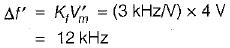Courses

# Angle Modulation

## 10 Questions MCQ Test Mock Tests Electronics & Communication Engineering GATE 2020 | Angle Modulation

Description
This mock test of Angle Modulation for Electronics and Communication Engineering (ECE) helps you for every Electronics and Communication Engineering (ECE) entrance exam. This contains 10 Multiple Choice Questions for Electronics and Communication Engineering (ECE) Angle Modulation (mcq) to study with solutions a complete question bank. The solved questions answers in this Angle Modulation quiz give you a good mix of easy questions and tough questions. Electronics and Communication Engineering (ECE) students definitely take this Angle Modulation exercise for a better result in the exam. You can find other Angle Modulation extra questions, long questions & short questions for Electronics and Communication Engineering (ECE) on EduRev as well by searching above.
QUESTION: 1

Solution:
QUESTION: 2

Solution:
QUESTION: 3

### The amount of frequency shift in FM is

Solution:

For FM, the frequency changes with the change in the amplitude of modulating signal value.

QUESTION: 4

Match List-l (Type of transmission) with List-l I (Applications) and select the correct answer using the codes given below the lists:
List-l
A. Wideband FM
B. AM
C. Narrowband FM
D. Vestigial sideband transmission
List-lI
2. Used for transmission of television signals
3. Used for high quality music transmission
4. Used for speech transmission (Police wireless, ambulance etc.)
Codes: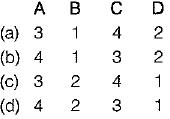Solution:
QUESTION: 5

According to Carson’s rule, the bandwidth of FM wave is given by (Aω is the frequency deviation and ωm the modulating frequency)

Solution:

According to Carson’s rule, the bandwidth of a single-tone wideband FM is
BW = 2(Δω + ωm)

QUESTION: 6

Consider the following statements associated with angle modulations:
1. In FM, the frequency deviation is proportional to modulating frequency.
2. In PM, the frequency deviation is independent of modulating signal.
3. Amplitude of both FM and PM are constant.
4. It is possible to receive FM on a PM receiver and vice-versa.
5. Signal to noise ratio of PM is better than that of FM.
Which of the statements given above are correct?

Solution:

• In FM, the frequency deviation is proportional only to the amplitude variation of the modulating signal and it is independent of modulating frequency fm. Hence, statement-1 is not correct.
• In PM, the frequency deviation increases with increase in modulating frequency fm and decreases with decrease in modulating frequency. Thus, frequency deviation in PM is proportional to modulating frequency. Hence, statement-2 is false.
• Statement-3 is correct.
• it is possible to receive FM on a PM receiver and PM on a FM receiver. Hence, statement-4 is correct.
• Signal to noise ratio of PM is inferior to that in FM. Hence, statement-5 is not correct.

QUESTION: 7

We can obtain PM from frequency modulator by passing the modulating signal through a

Solution:

A PM wave can be generated using a frequency modulator as shown in figure below: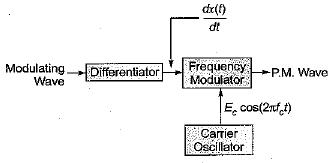QUESTION: 8

Match List-l (Type of modulation) with List-II (Noise immunity) and select the correct answer using the codes given below the lists: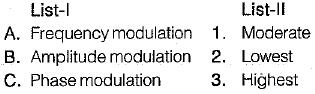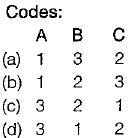Solution:

The noise immunity of FM is better than AM and PM while the noise immunity of PM is better than AM but worse than FM.

QUESTION: 9

A FM wave is represented by the following equation:
v = 10 sin [5 x 10B t + 4 sin 1250 t]
Its maximum deviation is around.

Solution:

Given, v = 10sin [5 x 108t + 4 sin 1250 t]
Comparing the above equation with the standard for FM given by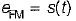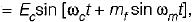we have modulating frequency,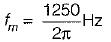and modulation index,
mf = 4
∴ Maximum deviation,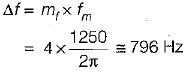QUESTION: 10

In a FM system, the modulating frequency fm = 1 kHz, the modulating voltage Vm= 2 volt and the deviation is 6 kHz. If the modulating voltage is raised to 4 volt then what is the new deviation?

Solution:

Given,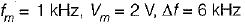We know that,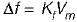or,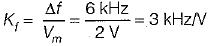When, V'm = 4 V, the new deviation in frequency is